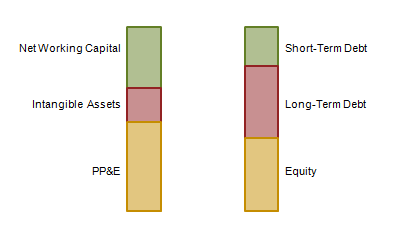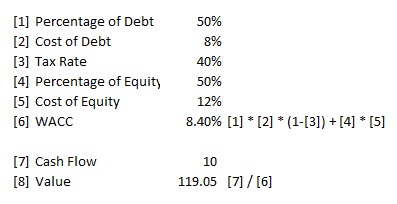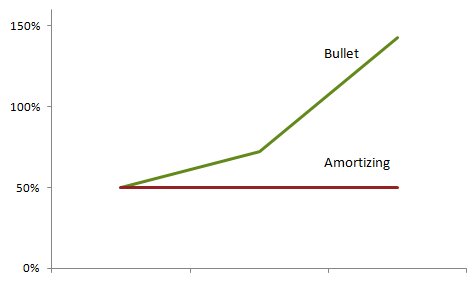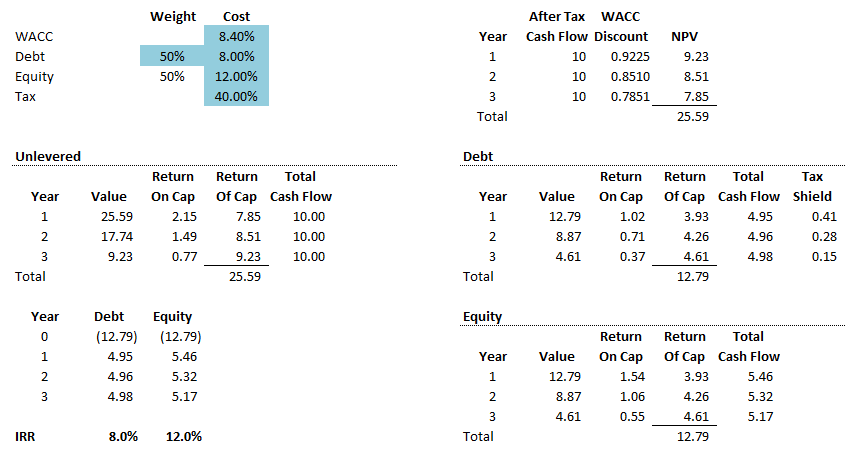## Tuesday, March 11, 2014

### Is My Weighted Average Cost of Capital WACC - y?

There are a lot of places where we can get the basic formula for a firm's Weighted Average Cost of Capital (often abbreviated as WACC).

Once we have calculated this figure, it can be employed in a number of settings - investment valuation, performance evaluation, industry comparison, etc.

Yet what is often not easy to find are the issues that arise during this employment - either nuances present in its calculation or the traps that can occur if we rotely employ it thereafter.

What Is Capital Structure?

We use the term "Capital Structure" to refer to the variety of instruments the firm has used to finance itself - short-term debt, long-term debt, preferred equity, common stock, options, warrants, etc.

These items are on the right side of the balance sheet. The left side of the balance sheet has assets. If we net the liabilities of items that are not investments (i.e. providers of these funds do not expect a return, such as Accounts Payable), then our balance sheet will show how these assets have been financed by the firm (see "How to Calculate ROIC" for an example of this netting).

Figure A
Capital StructureOur balance sheet will provide information like that shown in Figure A - it tells us what classes of assets the firm has and what instruments have been used to finance them.

The money the firm has received for each of these financial instruments comes from investors. In this context we define investors as those seeking a return for the funds they have provided. Banks and bond holders require interest, preferred equity holders require dividends, and common stock holders may require dividends and want to sell their shares at a higher price than what they paid for them.

Each of these investors has a different expectation about what they will receive. For example, a short-term debt investor will generally expect a lower interest rate than a long-term debt investor.

These "return expectations" are in large part driven by the market. We'd all love to see the stock we own quadruple in value over the next year, but it would be unrealistic to expect this result.

Opportunity Cost

The fact that an investor's expected return is driven by the market is due to the concept of opportunity cost.

Opportunity cost reflects the fact that we cannot have everything, and in order to get something we must give up something else. For a personal example, if we decide to go to the movies tonight, then we are not going to play soccer, have a long leisurely dinner, practice guitar, etc. during that time instead.

Financially, if we invest ♢ 10 (for new readers, the symbol ♢ stands for Treasury Cafe Monetary Units, or TCMU's, freely exchangable into any currency at any rate of your choosing) in X, then we cannot invest it in Y.

Thus Y "sets the bar" for X. If we would expect to make 10% per year investing in Y, then we need to do slightly better to choose X instead - perhaps 10.000001%.

The caveat on this is that the cost of capital is evaluated against investments with the same level of risk. We do not evaluate X against Z if Z is a "risk free" instrument and X is a risky one.

Weighted Average Cost of Capital I

So far we have established that a firm has a capital structure, made up of a number of financial instruments, and that each of these instruments has a cost of capital associated with it.

Figure B
Weighted Average Cost of Capital FormulaThe Weighted Average Cost of Capital is the proportional sum of the different instrument's in our firm's capital structure's cost.

Generically, absent other factors, it can be computed using the formula in Figure B.

Note that the formula in Figure B is hardly ever used in practice for the simple reason that, absent other factors, the weighted average cost of capital for the different financial instruments must equal the cost of capital of the firm as if it were funded with only equity. This is the Nobel prize winning Modigliani - Miller theorem.

For example, let's say that Joe's Agricultural Empire, LLC is in a business where the level of that risk is priced at 10% in the market. In other words, assets with the same level of risk are going for 10%, and therefore investor's require a similar rate of return for Joe's.

Suppose that Joe's produces ♢ 10 of cash flow for investors per year, and is expected to do so forever. We can use the dividend discount formula to calculate the equity value of Joe's at ♢ 100 (10/0.1).

Now imagine that we issue a financial instrument that's worth 50% of the value for Joe's, and the market prices the risk of this security at 8%. Why does the market price this differently? Because the risk characteristics of this security are different than the underlying assets.

It could be that it has a priority claim on the cash flow, meaning these investors get paid first, before the equity investors, so in the year's where the expected cash flow of ♢ 10 is not met, they get a bigger slice of the pie. Or it could be that the payout to these investors is protected from some of the risks Joe's faces, such as market prices (perhaps for a cost input or revenue generating factor).

Figure C
Weighted Average Cost of Capital FormulaAgain using the dividend discount formula, after using algebra to rearrange it, the cash flow to these investors is ♢ 4 (4/.08 = 50, which is 50% of the value as stipulated).

The key point for the pricing is that, for the market to price this instrument differently, there needs to be a reason for it related to risk. If the opportunity cost for this instrument is 8%, this instrument has a different risk characteristic than Joe's as a whole to make it that way.

The equity investors will get the remaining ♢ 6 of the expected cash flow, and the value of their investment is ♢ 50 (half the firm's value as stipulated), so their opportunity cost is now 12%. Figure C shows the calculations for this example.

Again, the reason the equity is priced differently is related to risk. Recall that some of the risks for the new instrument's investors was 'taken off the table', resulting in their pricing the new instrument at 8%. Yet, the remaining risk does not go away. It therefore is now disproportionately borne by the equity holder. Thus, higher level of risk, higher level of return required.

We now have a capital structure of 2 instruments, one at 8% and one at 12%, each representing 50% of the firm value. The weighted average cost of capital is 10% (50% * 8% + 50% * 12%), the same as before!

This leads to the Modigliani-Miller conclusion: how the firm is financed does not matter! We can think of it like squishing a balloon - if one part is less the other part becomes more. The volume of air in the balloon is the same, it is merely distributed differently. The same for the risks in the firm and how they are shared by investors.

The key takeaway from this is one of simple common sense: there is no such thing as a free lunch.

Weighted Average Cost of Capital II

The natural question to ask after this exercise is "why bother"? If the parts equal the whole, why bother breaking it down into parts when all that happens is that we get back to the same place? If financing doesn't really matter, let's keep it simple.

The key phrase from the last section is "absent other factors". There are other factors.

The biggest of these is taxes. Because interest is a deductible business expense, financing all of a sudden can drive value through the creation of the so-called "Tax Shield".

Figure D
Weighted Average Cost of Capital FormulaFigure D shows the value of the two components shown in Figure C with the addition of the interest expense deductibility. The example uses 40% as the assumed tax rate. Ultimately, financing with 50% debt adds about ♢ 13 of value to the firm through this deductibility.

Notice that the final capital structure is no longer 50% for both of the securities - the one is worth more than the other.

We can get back to 50% if we iterated through the valuation a few times. Set debt at 50% of ♢ 113, see how close we get to 50% with the new tax shield, adjust, and repeat again and again.

Figure E
Weighted Average Cost of Capital FormulaThe other way this problem is solved is to modify our Weigted Average Cost of Capital equation to that shown in Figure E.

In this figure we have added a second term to the formula to deal with instruments that generate tax shields. If an instrument does not generate tax shields, it is evaluated by the first term in the equation. If it does, it is evaluated by the second term.

Figure F
WACC Example CalulationWe can now go back to our Joe's Agricultural Empire LLC example. Figure F shows that by using our new formula, we calculate a weighted average cost of capital of 8.4%. Using this rate in our dividend valuation model, we arrive at a value of a little more than ♢ 119 for Joe's.

The beauty of the WACC approach is that we have captured the fact of tax shield generation in the denominator through the (1-t) term, so in the numerator we use the cash flow from the example before (called "unlevered" because they do not account for debt). We do not have to go through the effort of modeling the cash flows of all the various instruments in order to value the company, the WACC has done this all for us!

Simplicity has its benefits!

And it also has its drawbacks.

Problems with WACC

Using the Weighted Average Cost of Capital makes a number of assumptions.

Figure G
Term Structure TrapGiven the term structure in the upper portion, the payments in the first column of the lower portion are discounted in two ways, the first using the term structure and the second using the Year 3 debt rate. This results in an error of slightly more than 4% in valuation.

First, it assumes that rates are constant through the period we are considering. This is hardly ever the case in reality. Interest rates generally exhibit a "term structure", they vary depending on how long the debt will be outstanding. If we are going to pay off debt in 1 year, this rate is almost always lower than if we are going to wait 30 years to pay it off.

The general approach most take is to use the debt rate that reflects the length of time we are considering. If we need to use our WACC to value a 10-year payment stream, we will use the 10-year debt rate. If its 20 years, we will use a 20 year rate, and so on.

Figure G shows an example of this shortcut and how it results in a mis-valuation of the payments. We use the 3-year debt rate to value 3 years worth of cash flows. Because the cash flows are "lumpy" (not the same in every year), the valuation of them are driven more by the shorter term rates than the 3 year rates.

The solution to the above would be to use the actual term structure, weighted in accordance with the cash flows being evaluated by the WACC. Yet this can be difficult to do. Most companies do not have enough debt outstanding to establish a term structure on their own. In addition, there are points in the "term structure" spectrum which are more liquid than others - generally maturity related, such as 5 years, 10 years 20 years - which can impact pricing. There is less activity off these cycles, so if we have a 13 year cash flow stream it can be difficult to establish a term structure.

Awareness of this dynamic can be critical. If a company has used the WACC calculation to establish a "hurdle rate", which is the rate at which all investments will be evaluated, and has used long term debt rates to do so, then the business unit manager who has a 1-year project may be at a disadvantage, as their hurdle rate would be much lower had the WACC been calculated according to their shorter time horizon. This places the firm in a position where they will reject short term projects that would have in fact added value to undertake.

A second issue is the underlying assumption that the capital structure is constant throughout the term. Because we have calculated the value of tax shields and placed this cost of capital into the WACC, when this rate is used to discount cash flows the capital structure making up the WACC is assumed to be in place at that point in time.

So if we have a 13 year project we are valuing using our WACC, we are assuming that the capital structure (say the 50-50 in our Joe's eample earlier) is in place the entire time.

This again diverges from reality for a number of reasons.

First, capital structure is fluid, yet not generally thought of in that way. When we want to compare our capital structure to others, or earlier periods in our history, we generally use the balance sheet to obtain these numbers. The balance sheet is a "snapshot in time" portrayal of the assets and liabilities of the firm on a certain date. Yet, the assets and liabilities at a quarter end (the time usually for which balance sheets are prepared) may bear a different relation to the assets and liabilities in mid-month.

Figure H
Debt Capitalization ComparisonSecond, a lot of the debt companies issue have "bullet" maturities, meaning all the principal is due at the end of the debt term in one lump sum payment. This will lead to the capital structure being uneven.

Figure H shows the debt percentage of a project's capital structure for an amortizing debt instrument and a bullet debt instrument. The bullet debt instrument is consistently higher than 50% of the capital structure for all years except for the first.

Figure's I and J show the detail behind this example. Note that for the amortizing debt case in Figure I, the respective internal rates of return (IRR) for both the debt and equity match their cost of capital as used in the WACC formula. In Figure J, they do not.

Figure I
Financed With Amortizing DebtCost of capital assumptions in the top left. Valuation of the cash flows using this are in the top right. The distribution of the cash flows for return on capital and return of capital are in the middle left portion. The distribution of cash flows for the debt and equity portions are on the right. An internal rate of return comparison is on the bottom left.
Figure J
Financed With Bullet DebtCost of capital assumptions in the top left. Valuation of the cash flows using this are in the top right. The distribution of the cash flows for return on capital and return of capital are in the middle left portion. The distribution of cash flows for the debt and equity portions are on the right. An internal rate of return comparison is on the bottom left.
Figure K
Perpetuity FinancingThe Figure I and Figure J comparison highlights the fact that risk is impacted by how the debt payments are structured. In Figure I, the debt is amortized in proportion to the value 'consumed' by the project during that period of time. In Figure J, it all comes due at the end of the project, which means that equity holders have received their returns earlier, and actually have to contribute amounts in the final year to pay off the debt. This places the debt holders in a much more risky position than the ones in Figure I.

Figure K shows the case when the cash flows to be valued will go on forever (known as a perpetuity). In this instance, we can value the cash flows using the WACC or separately as debt and equity and arrive at the same value. This occurs because we have not violated the constant capital structure condition.

Another common problem occurs when we need to analyze a new project or investment. Since the WACC is driven by opportunity cost, our new project or investment will require a different WACC if the risk level is different than our other assets.

Going back to our Joe's example, we have a WACC of 8.4%. Let's say the company is evaluating whether it should buy some trucks to transport all their products around. Using the WACC calculated earlier will not be appropriate, because that was based on the opportunity cost of the agriculture business, not the trucking business. Owning and operating a fleet of trucks has an entirely different risk profile, and therefore requires a different WACC.

A shortcut often used is to use the face value of debt as a proxy for the market value. This is due to the fact that market values of debt can be difficult to determine. Debt securities are not traded everyday, and there is no end of the day ticker to establish value.

Sometimes face value is a 'good enough' approximation, but sometimes it is not. In Figure K, our WACC was established on 8% debt. If interest rates have gone down to 6% since that debt was issued, it will now be worth ♢ 79.37 rather than ♢ 59.52, a 33% difference!

What You Can Do

While the WACC calculation, as shown through these examples, has its fair share of warts, it nevertheless still has benefits.

It's a useful data point if taken in moderation - estimating our Weighted Average Cost of Capital provides a metric for us to refer to when valuing projects and opportunities, or for evaluating performance. This is better than nothing. The thing we need to remember is that it is not a 'be all, end all' calculation, but one piece of information among many.

Triangulate the WACC - calculating our WACC using different assumptions (changing the debt terms for instance) gives us a sense of the range of the measure. So while we may not be entirely confident that our 'true' cost of capital is 8.46731%, we may establish that it is likely to be somewhere between 8% and 10%. Another way we might establish a range is to calculate it for other firms that are similar to ours.

Key Takeaways

The Weighted Average Cost of Capital is commonly used to value a series of cash flows. However, it is prone to error because the underlying assumptions often do not match reality and/or the shortcuts applied in practice distort its value. Because of this, one should consider this calculation to be an approximation or indication rather than an absolutely correct value.

You May Also Like
Questions
::When was the last time you applied the WACC in an analysis?
::How many of the assumptions and shortcuts mentioned have you seen practiced?
::What other factors cause imprecision in the WACC calculation?

Add to the discussion with your thoughts, comments, questions and feedback! Please share Treasury Café with others. Thank you!